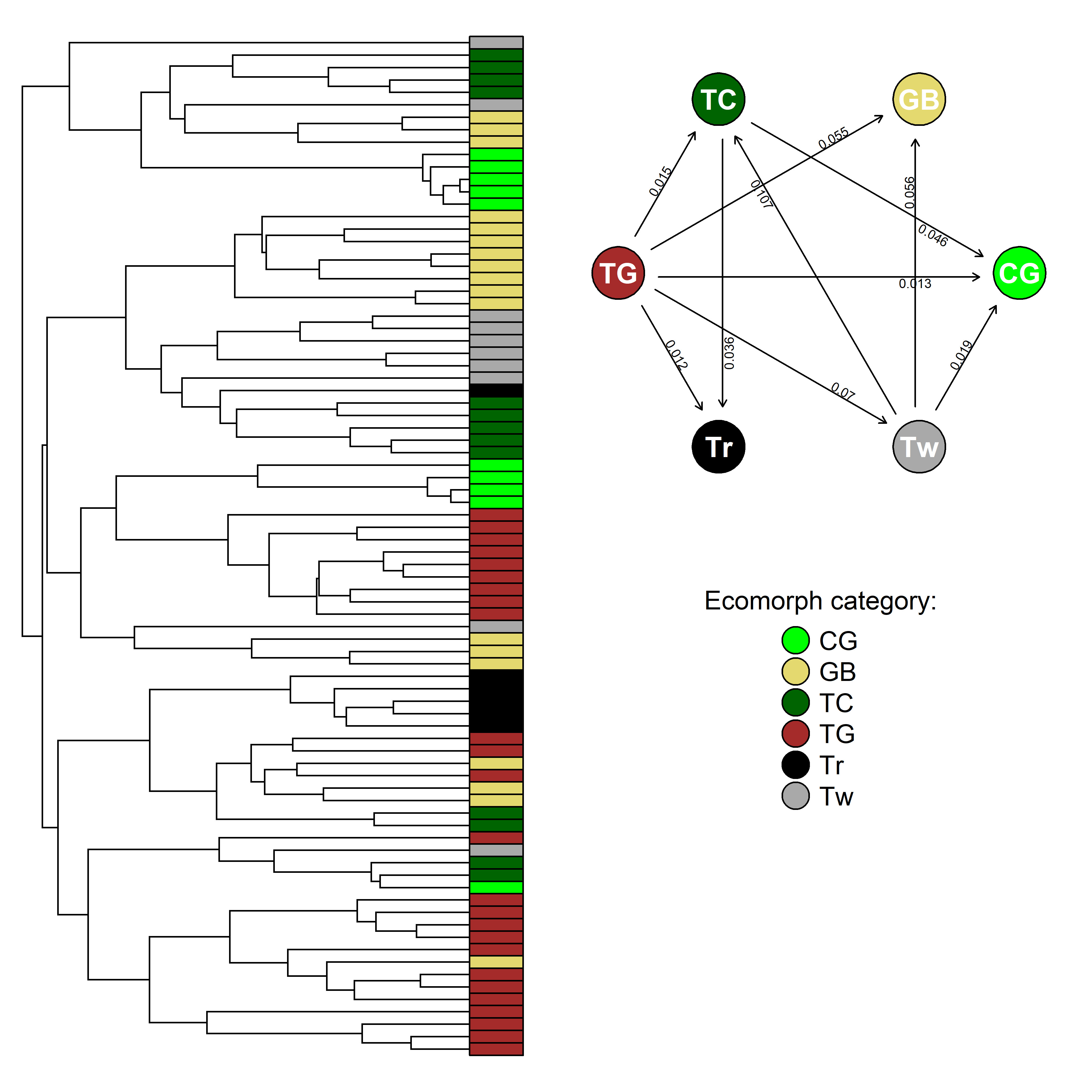## Sunday, September 27, 2020

### Customizing a plotted Mk discrete character evolution model

Over the past few days, I've been gradually documenting some of the new features in the latest phytools CRAN update (e.g., 1, 2, 3, 4).

Here's another small one… the S3 `plot` method, now `plot.Qmatrix` - used internally by all the `plot` methods for the `"fitMk"` object class (as well as the `"gfit"` object class from `geiger::fitDiscrete`) now invisibly returns the coordinates of the vertices of the graphed model to the user.

Here's a simple example using 'ecomorph' (a discrete character that describes different habitat specialists in Anolis lizards).

``````library(phytools)
``````

Now let's get our tree and data. We'll use the data object `anoletree` - but as this object is of class `"simmap"`, we'll actually first convert it to a simple `"phylo"` object.

``````data(anoletree)
anolis.tree<-as.phylo(anoletree)
ecomorph<-as.factor(getStates(anoletree,"tips"))
``````

Now let's fit an 'all-rates-different' (`"ARD"`) model to our data.

``````fitARD<-fitMk(anolis.tree,ecomorph,model="ARD",
pi="fitzjohn")
print(fitARD,digits=4)
``````
``````## Object of class "fitMk".
##
## Fitted (or set) value of Q:
##        CG     GB      TC      TG     Tr      Tw
## CG 0.0000 0.0000  0.0000  0.0000 0.0000  0.0000
## GB 0.0000 0.0000  0.0000  0.0000 0.0000  0.0000
## TC 0.0463 0.0000 -0.0825  0.0000 0.0362  0.0000
## TG 0.0130 0.0554  0.0151 -0.1653 0.0121  0.0697
## Tr 0.0000 0.0000  0.0000  0.0000 0.0000  0.0000
## Tw 0.0188 0.0558  0.1069  0.0000 0.0000 -0.1815
##
## Fitted (or set) value of pi:
## CG GB TC TG Tr Tw
##  0  0  0  1  0  0
## due to treating the root prior as (a) nuisance.
##
## Log-likelihood: -68.2146
##
## Optimization method used was "nlminb"
``````

Lastly, let's create our plot. Since the `plot` method for our object returns the vertices of the graph, we'll use the plotrix R package to add circles at each vertex.

``````layout(matrix(c(3,1,3,2),2,2,byrow=TRUE))
cols<-setNames(c("green","#E4D96F","darkgreen",
"brown","black","darkgrey"),
c("CG","GB","TC","TG","Tr","Tw"))
xy<-plot(fitARD,show.zeros=FALSE,spacer=0.2,
mar=rep(0.5,4))
library(plotrix)
invisible(mapply(draw.circle,xy\$x,xy\$y,col=cols,
text(xy\$x,xy\$y,xy\$states,cex=1.3,col="white",
font=2)
plot(NA,xlim=c(0,1),ylim=c(0,1),bty="n",axes=FALSE)
legend(0.25,1,xy\$states,cex=1.2,
pch=21,pt.cex=2.8,pt.bg=cols,
title="Ecomorph category:",bty="n")
h<-max(nodeHeights(anolis.tree))
plotTree(anolis.tree,ftype="off",
xlim=c(0,1.12*h),lwd=1)
pp<-get("last_plot.phylo",envir=.PlotPhyloEnv)
cols<-cols[ecomorph[anolis.tree\$tip.label]]
for(i in 1:Ntip(anolis.tree))
polygon(c(h,1.12*h,1.12*h,h),
rep(pp\$yy[i],4)+c(-0.5,-0.5,0.5,0.5),
col=cols[i])
``````Maybe a different color scheme is in order, but I feel like you could just about publish that. Cool.

1.Very cool! can we scale the arrow width according to the transition rate?

1.Hi Div. I posted an example of how to do it here, but in the future I may add it as a feature of the plotting function. - Liam

2.Hi Liam, this is a nice customization of plots.
When applying this code to my own data, it returns the following error:

Error in mapply(draw.circle, xy\$x, xy\$y, col = cols, MoreArgs = list(radius = 0.13)) :
zero-length inputs cannot be mixed with those of non-zero length

Any thoughts on how I'd overcome this?
Best wishes.

1.Do you have the latest CRAN version of phytools? Also make sure that you have the plotrix package loaded.

Note: due to the very large amount of spam, all comments are now automatically submitted for moderation.